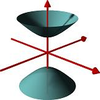#### You may also like### A Close Match

Can you massage the parameters of these curves to make them match as closely as possible?### Prime Counter

A short challenge concerning prime numbers.### The Right Volume

Can you rotate a curve to make a volume of 1?

# Common Divisor

##### Age 14 to 18Challenge Level

In this problem you will get the chance to discover some surprising results. Can you prove that they are always true?

Part A
Evaluate $1^3 - 1$, $2^3 - 2$, $3^3 - 3$ and $4^3 - 4$.
What is the largest integer that divides all of these?
Can you prove that it divides $n^3 - n$ for all positive integers $n$?

If a number is divisible by 6, which two prime numbers must it be divisible by?
To prove that the expression is always divisible by these two prime numbers, you could start by factorising $n^3 - n$.

Part B
Evaluate $1^5 - 1^3$, $2^5 - 2^3$, $3^5 - 3^3$ and $4^5 - 4^3$.
What is the largest integer that divides all of these?
Can you prove that it divides $n^5 - n^3$ for all positive integers $n$?

Work out which numbers you need to be able to divide by.
Factorising the general expression might be helpful again.
You can consider the cases $n$ even ($n=2k$) and $n$ odd ($n=2k+1$) separately.

Part C
Find the largest integer which divides every member of the following sequence: $$1^5-1,\ 2^5-2,\ 3^5-3,\cdots\, n^5-n.$$

Work out which prime numbers you need to be able to divide by.
Factorise the expression.  Can you show that the expression can be divided by these prime numbers?
You can consider different cases, perhaps starting with $n$ being a multiple of 5 $(n=5k)$.

Part D
Show that $2^{2n} -1$ can always be divided by three.

You can try some values of $n$ and see what happens.
You can consider the expression $2^n$ and what this can be divided by.